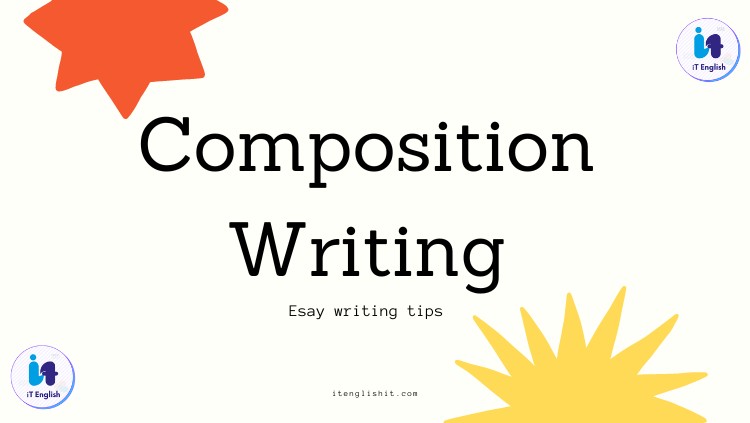# Essay/Composition writing tips for examinationA good way to approach an essay is to envision it as a Five Part  project. An essay is made up of the Introduction, Three main points  (the body), and the Conclusion. So it looks like this:

1. Introduction
2. Point One
3. Point Two
4. Point Three
5. Conclusion

Of course depending on the length and breadth of your paper you  may have more than three main points. However by using this structure  it will make envisioning your paper easier.

Introduction

An Introduction should answer three questions

1. What  am I talking about in this paper?
By answering this question  you let the reader know what the subject of the paper is. For  example, if your paper were about a particular book, your answer to  this question would give the title, author, and any other necessary  information.

2. How am I going to talk about it?
This  is where you let the reader know how your paper is organized. Here  you very briefly introduce your main points or the evidence that will  prove your point.

3. What am I going to prove in this paper?
This is the dreaded THESIS STATEMENT. The thesis is usually the last sentence in the first paragraph and it clearly states the  argument or point you are making in your paper.

Body

The Body consists of everything between your intro and conclusion and it is where you discuss your three main points. A good  starting place is to envision that each point is a separate paragraph  (or in a long paper each point might be a section). In each paragraph  you:

· Give supporting evidence (this is where quotes  go!)
· Explain how the point and evidence relate to  your thesis
The whole point of each paragraph is to relate your point to your thesis, but it helps to spell it out clearly in at least one sentence of the paragraph.

Conclusion

Basically, the conclusion restates the introduction. So just reiterate  questions 1, 2, and 3. It is also helpful to trace your argument as  you made it within the essay. A good way to do this is to create a  proof that might look something like this:

POINT  ONE+POINT TWO+POINT THREE=THESIS

OR

POINT ONE leads to  POINT TWO which leads to POINT THREE therefore THESIS is true!

So, when planning your essay consider this format:

I. Introduction
Subject
Main Points
Thesis

II. Point One
Intro and explanation of point
Evidence
How point relates to thesis

III. Point Two

A. Intro and explanation of point
Evidence
How point relates to thesis

IV. Point Three
Intro and explanation of point
Evidence
How point relates to thesis

V. Conclusion
Restate subject
Summarize Main Points

Restate Thesis

(B and C can be combined into the proof)
Organization in a paper is important not only because it makes the paper easier to write, it also guides the reader through the paper. A clearly organized paper will better hold the reader’s interest and convince them that your thesis is valid!